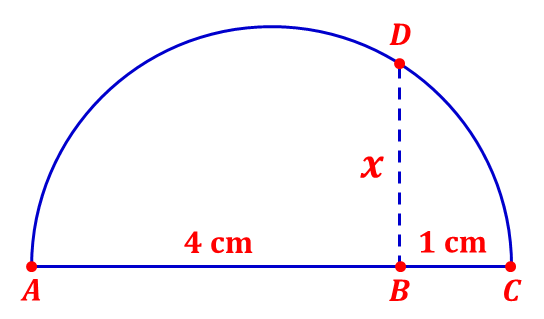# Segment in Semicircle

Geometry Level 1The picture above is a semicircle with diameter $\overline{AC},$ and $\overline{AC}$ is perpendicular to $\overline{BD}.$ It is known that $AB=4\text{ cm}$ and $BC=1\text{ cm}.$ If $BD=x,$ find $x.$

×